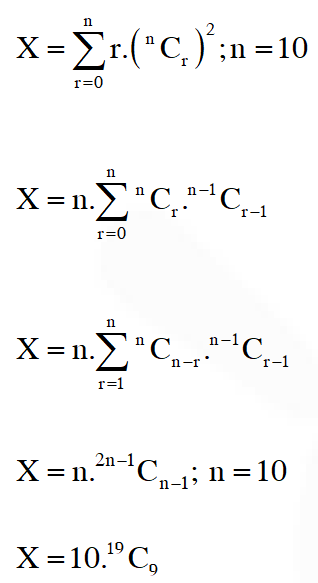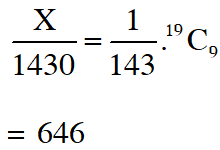# Binomial Theorem - JEE Advanced Previous Year Questions with Solutions

JEE Advanced Previous Year Questions of Math with Solutions are available at eSaral. Practicing JEE Advanced Previous Year Papers Questions of mathematics will help the JEE aspirants in realizing the question pattern as well as help in analyzing weak & strong areas. eSaral helps the students in clearing and understanding each topic in a better way. eSaral also provides complete chapter-wise notes of Class 11th and 12th both for all subjects. Besides this, eSaral also offers NCERT Solutions, Previous year questions for JEE Main and Advance, Practice questions, Test Series for JEE Main, JEE Advanced and NEET, Important questions of Physics, Chemistry, Math, and Biology and many more. Download eSaral app for free study material and video tutorials.
Q. For $\mathrm{r}=0,1, \ldots, 10,$ let $\mathrm{A}_{\mathrm{r}}, \mathrm{B}_{\mathrm{r}}$ and $\mathrm{C}_{\mathrm{r}}$ denote, respectively, the coefficient of $\mathrm{x}^{\mathrm{r}}$ in the expansions of $(1+\mathrm{x})^{10},(1+\mathrm{x})^{20}$ and $(1+\mathrm{x})^{30} .$ Then $\sum_{\mathrm{r}=1}^{10} \mathrm{A}_{\mathrm{r}}\left(\mathrm{B}_{10} \mathrm{B}_{\mathrm{r}}-\mathrm{C}_{10} \mathrm{A}_{\mathrm{r}}\right)$ is equal to $-\quad (A)$\mathrm{B}_{10}-\mathrm{C}_{10}$(B)$\mathrm{A}_{10}\left(\mathrm{B}_{10}^{2}-\mathrm{C}_{10} \mathrm{A}_{10}\right)$(C) 0 (D)$\mathrm{C}_{10}-\mathrm{B}_{10}$[JEE 2010, 5] Ans. (D)$\mathrm{A}_{\mathrm{r}}=^{10} \mathrm{C}_{\mathrm{r}}, \quad \mathrm{B}_{\mathrm{r}}=^{20} \mathrm{C}_{\mathrm{r}}, \mathrm{C}_{\mathrm{r}}=^{30} \mathrm{C}_{\mathrm{r}}\sum_{\mathrm{r}=1}^{10}\left(^{20} \mathrm{C}_{10}^{10} \mathrm{C}_{\mathrm{r}}^{20} \mathrm{C}_{\mathrm{r}}-^{30} \mathrm{C}_{10}\left(^{10} \mathrm{C}_{\mathrm{r}}\right)^{2}\right)=^{20} \mathrm{C}_{10}\left(^{10} \mathrm{C}_{1}^{20} \mathrm{C}_{1}+^{10} \mathrm{C}_{2}^{20} \mathrm{C}_{2}+\ldots .+^{10} \mathrm{C}_{10}^{20}\right)=^{20} \mathrm{C}_{10}\left(^{30} \mathrm{C}_{10}^{2}+1\right)-^{30} \mathrm{C}_{10}\left(^{20} \mathrm{C}_{10}-1\right)=^{20} \mathrm{C}_{10}-^{20} \mathrm{C}_{10}=\mathrm{C}_{10}-\mathrm{B}_{10}$Q. The coefficients of three consecutive terms of$(1+x)^{n+5}$are in the ratio$5: 10: 14 .$Then$\begin{array}{lll}{\text { n }=} & {\text { [JEE (Advanced) } 2013,4 \mathrm{M},-1 \mathrm{M}}\end{array}$[JEE (Advanced) 2013, 4M, –1M] Ans. 6 Let the three consecutive terms be$^{\mathrm{n}+5} \mathrm{C}_{\mathrm{r}-1},^{\mathrm{n}+5} \mathrm{C}_{\mathrm{r}},^{\mathrm{n}+5} \mathrm{C}_{\mathrm{r}+1}\therefore \quad \frac{^{n+5} C_{r-1}}{^{n+5} C_{r}}=\frac{1}{2} \quad \Rightarrow \quad \frac{r}{n-r+6}=\frac{1}{2}\Rightarrow \quad \mathrm{n}=3 \mathrm{r}-6$........(1) Also,$\frac{n+5 \mathrm{C}_{\mathrm{r}}}{^{\mathrm{n}+5} \mathrm{C}_{\mathrm{r}+1}}=\frac{5}{7} \Rightarrow \frac{\mathrm{r}+1}{\mathrm{n}-\mathrm{r}+5}=\frac{5}{7}$.$\Rightarrow$12r = 5n + 18 ........(2) Solving (1) and (2), we get n = 6 Q. Coefficient of$x^{11}$in the expansion of$\left(1+x^{2}\right)^{4}\left(1+x^{3}\right)^{7}\left(1+x^{4}\right)^{12}$is - [JEE(Advanced)-2014, 3(–1)] Ans. (C) Coefficient of$\mathrm{x}^{11}$in$\left(1+\mathrm{x}^{2}\right)^{4}\left(1+\mathrm{x}^{3}\right)^{7}\left(1+\mathrm{x}^{4}\right)^{12}=^{4} \mathrm{C}_{0} \cdot^{7} \mathrm{C}_{1}^{12} \mathrm{C}_{2}+^{4} \mathrm{C}_{1} \cdot^{7} \mathrm{C}_{3}^{12} \mathrm{C}_{0}+^{4} \mathrm{C}_{2} \cdot^{7} \mathrm{C}_{1} \cdot^{12} \mathrm{C}_{1}+^{4} \mathrm{C}_{4} \cdot^{7} \mathrm{C}_{1} \cdot^{12} \mathrm{C}_{0}=462+140+504+7=1113$Q. The coefficient of$x^{9}$in the expansion of$(1+x)\left(1+x^{2}\right)\left(1+x^{3}\right) \ldots\left(1+x^{100}\right)$is [JEE 2015, 4M, –0M] Ans. 8 There are 8 product$1^{99} \mathrm{x}^{9}, 1^{98} \mathrm{x}^{8}, 1^{98} \mathrm{x}^{2} \mathrm{x}^{7}, 1^{98} \mathrm{x}^{3} \mathrm{x}^{6}, 1^{98} \mathrm{x}^{4} \mathrm{x}^{5}1^{97} \mathrm{x} \mathrm{x}^{2} \mathrm{x}^{6}, 1^{97} \mathrm{x}^{3} \mathrm{x}^{5}, 1^{97} \mathrm{x}^{2} \mathrm{x}^{3} \mathrm{x}^{4}$which generate$\mathrm{x}^{9}$so coeff. is 8 Q. Let$m$be the smallest positive integer such that the coefficient of$x^{2}$in the expansion of$(1+x)^{2}+(1+x)^{3}+\ldots \ldots+(1+x)^{49}+(1+m x)^{50}$is$(3 n+1)^{51} C_{3}$for some positive integer n. Then the value of$n$is [JEE (Advanced) 2016] Ans. 5 Coefficient of$x^{2}$in the expansion of$(1+x)^{2}+(1+x)^{3}+\ldots \ldots(1+x)^{49}+(1+m x)^{50}$is$^{2} C_{2}+^{3} C_{2}+\ldots \ldots^{49} C_{2}+^{50} C_{2} m^{2}=(3 n+1)^{51} C_{3}^{3} C_{3}+^{3} C_{2}+\ldots \ldots^{49} C_{2}+^{50} C_{2} m^{2}=(3 n+1)^{51} C_{3}\quad^{50} C_{3}+^{50} C_{2} m^{2}=(3 n+1)^{51} C_{3}\frac{50.49 .48}{6}+\frac{50.49}{2} \mathrm{m}^{2}=(3 \mathrm{n}+1) \frac{51.50 .49}{6}\mathrm{m}^{2}=51 \mathrm{n}+1$must be a perfect square$\Rightarrow \mathrm{n}=5$and$\mathrm{m}=16$Ans.$\Rightarrow 5$Q. Let$\mathrm{X}=\left(^{10} \mathrm{C}_{1}\right)^{2}+2\left(^{10} \mathrm{C}_{2}\right)^{2}+3\left(^{10} \mathrm{C}_{3}\right)^{2}+\ldots+10\left(^{10} \mathrm{C}_{10}\right)^{2},$where$^{10} \mathrm{C}_{\mathrm{r}^{\prime}}, \mathrm{r} \in\{1,2, \ldots, 10\}$denote binomial coefficients. Then, the value of$\frac{1}{1430} \mathrm{X}\$ is [JEE(Advanced)-2018, 3(0)]
Ans. 646lucky
Sept. 5, 2021, 9:58 p.m.
I clear my doubt ......
Issac Newton
May 2, 2021, 4:40 p.m.
Hey why does everyone look at me just like I have done gravitation and opticks? This is one of my finest works !!!
D
Feb. 1, 2021, 8:30 a.m.
Waste
Arun kumar thulla
Sept. 22, 2020, 3:04 p.m.
Sir awesome website 👏👏
Abhiram
Sept. 19, 2020, 2:19 p.m.
Awesome website
Vamshi
June 4, 2020, 12:34 p.m.
Sir please send the PDF of binomial theorem chapter and it's problems.🤲🤲
None
Free Study Material